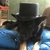## FANDOM

120 Pages# Keo5

## aka Tiger

• I live in America (Muh•reh•cuh)
• I am Flammable
•## 3 Mathematical Conundrums

December 24, 2011 by Keo5

I stubled across 3 false proofs, see if you can find the errors.

• X=Y
• X^2=X*Y
• X^2-Y^2=X*Y-Y^2
• (X+Y)*(X-Y)=Y*(X-Y)
• X+Y=Y
• 2Y=Y
• 2=1

• X=0.99999...
• 10X=9.99999...
• 10X-X=9.99999...-X
• 9X=9
• X=1
• 0.99999...=1

This one is a word problem. Imagine a square with sides of 4, and therefore a perimiter of 16. Cut corners, and the perimiter stays the same. See below for a visual.

• HHHHHHHHHH
• HHHHHHHHHH
• HHHHHHHHHH
• HHHHHHHHHH
• HHHHHHHHHH
• HHHHHHHHHH

The perimeter of the above shape is equal to the perimeter of the shape below. (_ equals negative space)

• ____HHHH____
• ____HHHH____
• HHHHHHHHHH
• HHHHHHHHHH
• ____HHHH____
• ____HHHH____

Repeat to infinity. Square becomes a circle

• 16=Pi*(2)^2

Therefore, Pi equals 4

That's all folks!

~₮iger~ :. Talk & Profile .: